# Conservation Of Linear Momentum

## Impluse And Momentum of Class 11

When the sum of the forces on an object is zero, the equation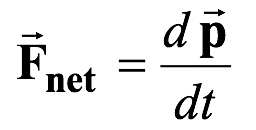tell us that the time derivative of momentum is zero.

That is,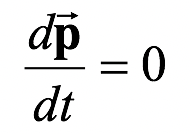This implies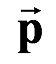= constant (9.8)

### Consequently, one can state a conservation law for momentum:

When the net force on a particle is zero, its momentum is constant.

The real utility of the momentum conservation concept comes about when it is applied to a collection of particles. For a system of particles, the total momentum is simply the vector sum of the momentum of each of the particles in the system. That is=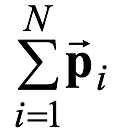(9.9)

Now consider the net force on a system of particles.

### There are two kinds of forces:

(i) Internal forces, resulting from the forces between the particles within the system, and

(ii) External forces, arising from the forces between the particles in the system and objects outside the system.

For example, consider a system of two blocks joined together with a spring. If the system is allowed to fall freely under gravity, then the gravitational force acting on each block is the external force and the spring force acting on each block is internal.

When we calculate the net force on a system of particles by performing the vector sum, then the summation of all the internal forces is zero.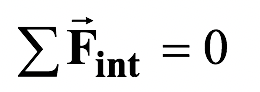Thus, equation (9.7) may be written as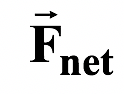=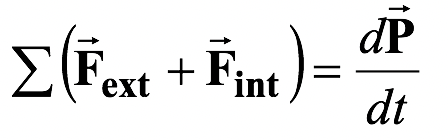or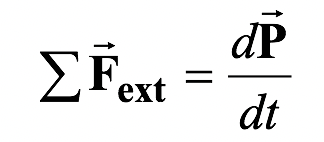(9.10)

Now, if the summation of external forces is zero, thenThus,= constant

### Law of Conservation of Momentum

In the absence of a net external force, the momentum of a system is conserved.

The conservation of momentum law can be used to relate the initial motion of particles within a system to the motion of those same particles sometime later. The law emphasizes the equality of momentum before and after something happens within the system.

Thus, the conservation law is usually written as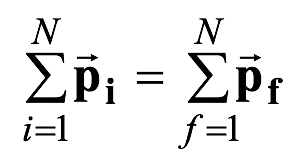(9.11)

 Example 9.6 Suppose that a bullet, of mass m = 10 g and speed u, is fired into a block of mass M = 2kg suspended as in figure. The bullet embeds in the block and raises it by a height H = 5 cm. (a)How can one determine u from H? (b)What is the thermal energy generated?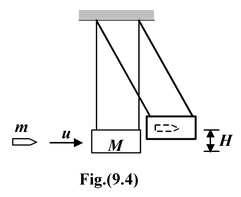Solution

If the collision occurs within a very short time interval, the ropes will remains essentially vertical as the bullet comes to rest. Thus, there will be no external horizontal force. We may apply the conservation of linear momentum along this direction.

mu = (m + M)V (i)

where V is the common velocity after the collision. Since the collision is completely inelastic part of the bullet’s initial kinetic energy, 1/2mu2, is converted into thermal energy. Only the kinetic energy of the bullet-block system that remains after the collision is available to raise the system by height H. From the conservation of mechanical energy we have

1/2(m + M)V2 = (m + M)gH(ii)

Hence, V = (2gH)1/2. Substituting this into equation (i), we find

u =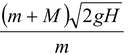Putting m = 10-2 kg; M = 2 kg; H = 0.05 m; g = 10m/s2

We get u =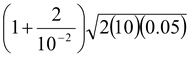oru = 201 m/s

(b)The kinetic energies before and after the collision are

Ki = 1/2mu2 = 202 J and Kf = 1/2(m + M)V2 = 1 J

The change in the kinetic energy as a result of the collision is –201 J. Virtually all the bullet’s kinetic energy is converted into thermal energy.